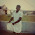# Class 7, Solutions of Ncert Cbse Mathematics | Chapter 3, Data Handling | NCERT Math Textbook Exercise 3.1 Solved

Class 7, CBSE Mathematics
Chapter 3, DATA HANDLING
Solutions of NCERT Math Textbook Exercise 3.1
Q1: Find the range of heights of any ten students of your class.
Solution:
Let the heights (in cm) of any 10 students of our class be 125, 127, 132, 133, 134, 136,138, 141, 144, 146.
=> Highest value among these observations = 146 cm
=> Lowest value among these observations = 125 cm
We know, Range = (Highest value − Lowest value)
= (146 − 125) cm
or, Range = 21 cm
Q2: Organize the following marks in a class assessment, in a tabular form.
4, 6, 7, 5, 3, 5, 4, 5, 2, 6, 2, 5, 1, 9, 6, 5, 8, 4, 6, 7
(i) Which number is the highest?
(ii) Which number is the lowest?
(iii) What is the range of the data?
(iv) Find the arithmetic mean.
Solution:
 Marks Tally marks Frequency 1 1 2 2 3 1 4 3 5 5 6 4 7 2 8 1 9 1
(i) Highest number = 9
(ii) Lowest number = 1
(iii) Range = (9 − 1) = 8
(iv) Sum of all the observations =
4 + 6 + 7 + 5 + 3 + 5 + 4 + 5 + 2 + 6 + 2 + 5 + 1 + 9 + 6 + 5 + 8 + 4 + 6 + 7
= 100
Total number of observations = 20

Q3: Find the mean of the first five whole numbers.
Solution:
First five whole numbers are 0, 1, 2, 3, and 4.

Therefore, the mean of first five whole numbers is 2.
Q4: A cricketer scores the following runs in eight innings:
58, 76, 40, 35, 46, 45, 0, 100.
Find the mean score.
Solution:
Runs scored by the cricketer = 58, 76, 40, 35, 46, 45, 0, and 100.

So, the mean score is 50.
Q5: Following table shows the points of each player scored in four games:
 Player Game 1 Game 2 Game 3 Game 4 A 14 16 10 10 B 0 8 6 4 C 8 11 Did not play 13
(i) Find the mean to determine A’s average number of points scored per game.
(ii) To find the mean number of points per game for C, would you divide the total points by 3 or by 4? Why?
(iii) B played in all the four games. How would you find the mean?
(iv) Who is the best performer?
Solution:
(i) A’s average number of points =

(ii) To find the mean number of points per game for C, we will divide the total points by 3 because C played 3 games.
(iii) Mean of B’s score =

(iv) The best performer will have the greatest average among all players. Now we find that the average of A is 12.5 which is more than that of B and C. Therefore, A is the best performer among these three.
Q6: The marks (out of 100) obtained by a group of students in a science test are 85, 76, 90, 85, 39, 48, 56, 95, 81 and 75. Find the:
(i) Highest and the lowest marks obtained by the students.
(ii) Range of the marks obtained.
(iii) Mean marks obtained by the group.
Solution:
First, let us arrange the marks obtained by the group of students in an ascending order as follows:
39, 48, 56, 75, 76, 81, 85, 85, 90, 95.
(i) Highest marks = 95 and
Lowest marks = 39
(ii) Range = (Highest value − Lowest value) = 95 − 39 = 56
(iii) Mean marks =

Q7: The enrollment in a school during six consecutive years was as follow:
1555, 1670, 1750, 2013, 2540, 2820
Find the mean enrollment of the school for this period.
Solution:
Mean enrollment of the school for this period =

Q8: The rainfall (in mm) in a city on 7 days of a certain week was recorded as follows:
 Days Rain fall (in mm) Monday 0.0 Tuesday 12.2 Wednesday 2.1 Thursday 0.0 Friday 20.5 Saturday 5.5 Sunday 1.0
(i) Find the range of the rainfall in the above data.
(ii) Find the mean rainfall for the week.
(iii) On how many days was the rainfall less than the mean rainfall.
Solution:
(i) Range = (20.5 − 0.0) mm = 20.5 mm
(ii) Mean rainfall for the week =

(iii) The rainfall for 5 days (i.e., Monday, Wednesday, Thursday, Saturday, Sunday) was less than the average rainfall.
Q9: The heights of 10 girls were measured in cm and the results are as follows:
135, 150, 139, 128, 151, 132, 146, 149, 143, 141
(i) What is the height of the tallest girl?
(ii) What is the height of the shortest girl?
(iii) What is the range of the data?
(iv) What is the mean height of the girls?
(v) How many girls have heights more than the mean height.
Solution:
Let us arrange the heights of 10 girls in an ascending order,
128, 132, 135, 139, 141, 143, 146, 149, 150, 151
(i) Height of the tallest girl = 151 cm
(ii) Height of the shortest girl = 128 cm
(iii) Range = (151 − 128) cm = 23 cm
(iv) Mean height =

(v) Total 5 girls have greater height the mean height (i.e., 141.4 cm) and the heights of these five girls are 143, 146, 149, 150, and 151 cm respectively.

1.2.